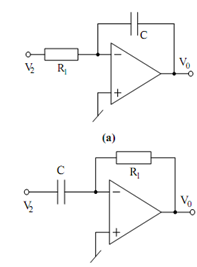## Inverting Integrator Assignment Help

Assignment Help: >> Basic Analog Circuits using Ideal Op-amps - Inverting Integrator

Inverting Integrator:

In Figure (a), if R is replaced by a capacitor (having transformed impedance 1/sC) one get the circuit of Figure (a) which is an inverting integrator.(b)

Figure: (a) Inverting Integrator (Miller Integrator); and (b) Inverting Differentiator

Replacing R2 in Eq. (7.55) by 1/ sC, the transfer function of the integrator of

Figure (a) is found to be

V 0 (S ) =- (1 / sC R 1 ) V  (S )

or in time domain,

V0 (t ) =-  1 /C R1 ∫ V2  (t ) dt

which represents an inverting integrator.